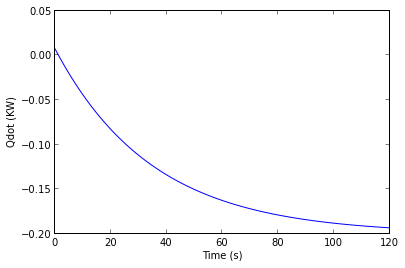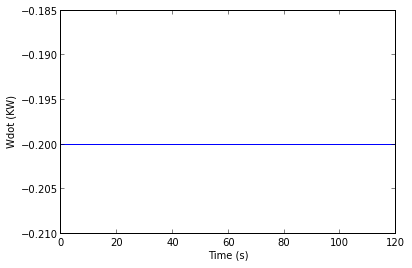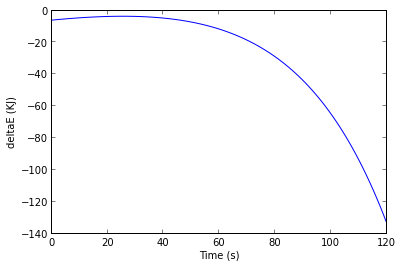# Chapter 2:- Energy and the First Law Of Thermodynamics¶

## Example 2.1 Page no-39

In :
# Given:-
p1 = 3*(10**5)                                # initial pressure of gas in pascal
v1 = 0.1                                      # initial volumme of gas in meter^3
v2 = 0.2                                      # final volume of gas in meter^3

# calculations
from scipy import integrate
import math
# Part (a) i.e. n=1.5
#constant = p1*(v1**n)                         # p*(v^n) = constant
constant1 = p1*(v1**1.5)
constant2 = p1*(v1**1)
constant3 = p1*(v1**0)
# function p
p1 = lambda v: constant1/(v**1.5)                   # expressing pressure as function of volume
p2 = lambda v: constant2/(v**1)
p3 = lambda v: constant3/(v**0)
# function [work1]
work1 = integrate.quad(p1,v1,v2)                   # integrating pdv from initial to final volume
w1 = work1/1000                             # divided by 1000 to convert to KJ
print 'The work done for n=1.5 in KJ is',round(w1,2)

#part(b) i.e. n = 1
w2 = work2/1000
print 'The work done for n=1 in KJ is',round(w2,2)

#part(c) i.e. n=0
w3 = work3/1000
print 'The work done for n=0 in KJ is',round(w3,2)

The work done for n=1.5 in KJ is 17.57
The work done for n=1 in KJ is 20.79
The work done for n=0 in KJ is 30.0

## Example 2.2 Page no-50

In :
# Given:-
p1 = 3*(10**5)                                  # initial pressure in pascal
v1 = 0.1                                        # initial volume in m3
v2 = 0.2                                        # initial volume in m3
m = 4.0                                         # mass of the gas in kg
deltau = -4.6                                   # change in specific internal energy in KJ/Kg

# Calculations
from scipy import integrate
import math
constant = p1*(v1**1.5)                           # p*(v^n) = constant

p = lambda v: constant/(v**1.5)                                # expressing pressure as function of volume

work = integrate.quad(p,v1,v2)                         # integrating pdv from initial to final volume
w=work/1000                              # divided by 1000 to convert to KJ

deltaU = m*deltau                           # change in internal energy in KJ
Q = deltaU + w                                # neglecting kinetic and potential energy changes

# Result
print 'net heat transfer for the process in KJ',round(Q,2)

net heat transfer for the process in KJ -0.83

## Example 2.3 Page no-52

In :
# Given:-
patm = 10**5                               # atmospheric pressure in pascal.
mp = 45.0                                  # mass of piston in Kg
A = 0.09                                   # face area of piston in m2
deltaV = 0.045                             # increment of the volume of air in m3
m = 0.27                                   # mass of air in kg
deltau = 42.0                              # specific internal energy increase of air in kJ/kg
g = 9.81                                   # local acceleration of gravity

# Part (a) i.e. air is system
# Calculations
p = (mp*g)/A + patm                         # constant pressure of air obtained from equilibrium of piston
w = (p*deltaV)/1000                         # work done in KJ
deltaU = m*deltau                           # internal energy change of air in KJ
Q = w + deltaU                              # applying first with air as system
# Result
print 'The answer given in book is incorrect.They have miscalculated deltaU.The correct heat transfer from resistor to air in KJ for air alone as system is: '
print round(Q,2)

# The answer given in book is incorrect. deltaU is incorrect in book.

# Part(b) i.e.  (air+piston) is system
# Calculations
wd = (patm*deltaV)/1000                      # work done in KJ
deltaz = (deltaV)/A                          # change in elevation of piston
deltaPE = (mp*g*deltaz)/1000                 # change in potential energy of piston in KJ
Qt = wd + deltaPE + deltaU                   # applying first law with air plus piston as system
# Result
print 'The answer given in book is incorrect.They have miscalculated deltaU.The correct heat transfer from resistor to air in KJ for air + piston as system is:'
print round(Qt,2)

The answer given in book is incorrect.They have miscalculated deltaU.The correct heat transfer from resistor to air in KJ for air alone as system is:
16.06
The answer given in book is incorrect.They have miscalculated deltaU.The correct heat transfer from resistor to air in KJ for air + piston as system is:
16.06

## Example 2.4 Page no-54

In :
# Given:-
w1dot = -60.0                # input work rate in KW
h = 0.171                    # heat transfer coefficient,unit in KW/m2 .K
A = 1.0                      # outer surface area of gearbox, unit in m2
Tb = 300.0                   # outer surface temperature in kelvin
Tf = 293.0                   # temperature of the sorrounding

# Calculations
Qdot = -h*A*(Tb-Tf);         # rate of energy transfer by heat
wdot = Qdot;                 # steady state energy equation
w2dot = wdot-w1dot;

# Results
print 'The heat transfer rate in KW is:\n\tQdot =  ',Qdot
print 'The power delivered through output shaft in KW is: = ',w2dot

The heat transfer rate in KW is:
Qdot =   -1.197
The power delivered through output shaft in KW is: =  58.803

## Example 2.5 Page no-55

In :
# Given:-
s=5*(10**-3)                       # measurement on a side in meter
wdot = -0.225                        # power input in watt
Tf = 293.0                           # coolant temprature in kelvin
h = 150.0                            # heat transfer coefficient in w/m2 k
A = s**2                            # surface area

# Calculation
Tb = ((-wdot/(h*A)) + Tf - 273)     # surface temperature in degree

# Result
print 'The surface temperature of the chip in degree celcius is:  ',Tb

The surface temperature of the chip in degree celcius is:   80.0

## Example 2.6 Page no-57

In :
%matplotlib inline

Welcome to pylab, a matplotlib-based Python environment [backend: module://IPython.zmq.pylab.backend_inline].
For more information, type 'help(pylab)'.
In :
# Given:-
omega = 100.0                      #motor rotation speed in rad/s
tau = 18.0                         #torque applied by shaft in N.m
Welecdot = -2.0                    #electric power input in KW

Wshaftdot = (tau*omega)/1000      #shaft work rate in KW
Wdot = Welecdot + Wshaftdot       #net work rate in KW

#function [Qdot]=f(t)
#Qdot = (-0.2)* [1-2**(-0.05*t)]

#function [Edot]=f1(t)               #function for rate of change of energy
#Edot = (-0.2)*[1-2**(-0.05*t)] - Wdot

#function [deltaE] =f2(t)            #function for change in energy
from sympy import *
x = symbols('x')             # a = 0.2 b = 0.05
f = (0.2)*(1-2**(0.05*(x))) - Wdot
f2 = integrate(f,x)
print f2

Qd = []
Wd = []
dltaE = []
from numpy import linspace
from pylab import plot, show
t = linspace(0,120,100);
for i in range(0,100):
Qd.append(i)
Wd.append(i)
dltaE.append(i)
Qd[i] =  (-0.2*(1-2**(-0.05*(120/99)*(i-1))))
Wd[i] = Wdot
dltaE[i] = -4.0*2**(0.05* (120/99)*(i-1))/log(2) + 0.4* (120/99)*(i-1)

plot(t,Qd)
xlabel("Time (s)")
ylabel("Qdot (KW)")
show()
plot(t,Wd)
xlabel("Time (s)")
ylabel("Wdot (KW)")
show()
plot(t,dltaE)
xlabel("Time (s)")
ylabel("deltaE (KJ)")
show()


-4.0*2**(0.05*x)/log(2) + 0.4*x